2019-03-22 13:44:27 qq_40093853 阅读数 214
• ###### Unity 值得看的500+ 技术内容列表

Unity3D是由Unity Technologies开发的一个让玩家轻松创建诸如三维视频游戏、建筑可视化、实时三维动画等类型互动内容的多平台的综合型游戏开发工具，是一个全面整合的专业游戏引擎。

# 使用效果

``````public class TestScript : MonoBehaviour,ISingleCoroutine
{
private void Awake()
{
this.StartSingleCoroutine(1, Tick1(1));
this.StartSingleCoroutine(2, Tick1(2));
this.StartSingleCoroutine(2, Tick1(3));

this.StartSingleCoroutine(3, Tick2());
this.StopSingleCoroutine(3);
}
private void OnDestroy()
{
this.StopAllSingleCoroutines();
}
IEnumerator Tick1(int i)
{
for (; ; )
{
Debug.Log("Howdy"+i);
yield return null;
}
}
IEnumerator Tick2()
{
for (; ; )
{
Debug.Log("Hello Thereeeee");
yield return null;
}
}
}
``````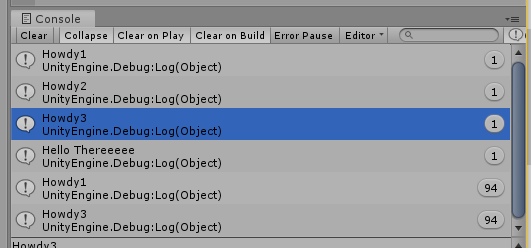# 思路原理

Update内遍历,满足条件则执行.

``````public class TestScript : MonoBehaviour
{
private void Awake()
{
Coroutine cor_temp;		//缓存
cor_temp = StartCoroutine(IETemp());		//创建
StopCoroutine(cor_temp);		//销毁
}

IEnumerator IETemp()
{
for (; ; )
{
Debug.Log("Howdy");
yield return null;
}
}
}
``````

# 代码实现

1.将所有携程集中到一个Monobehaviour单例中管理
2.需要使用携程的脚本继承接口并分配接口ID
3.通过接口从元函数创建与销毁携程

:
SingleTonMono 为个人使用的Mono单例模板类,初始化时创建实例

``````using System;
using System.Collections;
using System.Collections.Generic;
using UnityEngine;

public interface ISingleCoroutine //定义接口
{
}
class CoroutineManager : SingletonMono<CoroutineManager>	//管理器单例 调用时创建
{
internal static List<ISingleCoroutine> L_Target = new List<ISingleCoroutine>();
internal static Dictionary<int, Coroutine> Dic_Coroutines = new Dictionary<int, Coroutine>();
Dictionary<int, Coroutine> CoroutineDic = new Dictionary<int, Coroutine>();
internal static void QuestForRange(ISingleCoroutine target, ref int min, ref int max)	//接口请求携程ID范围
{
min = L_Target.FindIndex(p => p == target) * 1000;
max = min + 999;
}
internal static int QuestForIndex(ISingleCoroutine target, int index)		//携程请求ID
{
int targetIndex = 0;
if (!L_Target.Contains(target))

targetIndex += L_Target.FindIndex(p => p == target) * 1000;
targetIndex += index;
return targetIndex;
}
}
``````

``````public static class ISingleCoroutine_Extend
{
public static void StartSingleCoroutine(this ISingleCoroutine target, int index, IEnumerator numerator)	//开启单例携程
{
if (index < 0)
Debug.LogWarning(" Should Not Add Coroutine Index Which Below 0");

int targetIndex = CoroutineManager.QuestForIndex(target, index);
if (CoroutineManager.Dic_Coroutines.ContainsKey(targetIndex))
if (CoroutineManager.Dic_Coroutines[targetIndex] != null)
CoroutineManager.Instance.StopCoroutine(CoroutineManager.Dic_Coroutines[targetIndex]);
else

CoroutineManager.Dic_Coroutines[targetIndex] = CoroutineManager.Instance.StartCoroutine(numerator);
}
public static void StopSingleCoroutine(this ISingleCoroutine target, int index = 0)	//终止单例携程
{
int targetIndex = CoroutineManager.QuestForIndex(target, index);
if (CoroutineManager.Dic_Coroutines.ContainsKey(targetIndex) && CoroutineManager.Dic_Coroutines[targetIndex] != null)
CoroutineManager.Instance.StopCoroutine(CoroutineManager.Dic_Coroutines[targetIndex]);
}

public static void StopAllSingleCoroutines(this ISingleCoroutine target)		//终止单个接口申请的所有单例携程
{
int min=0, max = 0;
CoroutineManager.QuestForRange(target,ref min,ref max);
foreach (int index in CoroutineManager.Dic_Coroutines.Keys)
{
if (index >= min && index <= max)
CoroutineManager.Instance.StopCoroutine(CoroutineManager.Dic_Coroutines[index]);
}
}

public static void StopSingleCoroutines(this ISingleCoroutine target, params int[] indexes)		//终止多个携程
{
for (int i = 0; i < indexes.Length; i++)
StopSingleCoroutine(target, indexes[i]);
}
}
``````

# 未来优化

1.还未进行容错处理,如申请一个超过999的ID会出现ID冲突
2.用int作为携程ID索引不是很直观,可以采用枚举代替
3.能够在此基础上实现DoTween的效果(用Transform代替接口与单例ID并增加从元函数)

``````该文章只能提供思路,个人学疏才浅,无法保证是否会产生性能问题.
--StriteR 2019/3/22
``````

unity3d 创建携程 相关内容

2020-02-12 15:37:16 qq_42194657 阅读数 99
• ###### Unity 值得看的500+ 技术内容列表

Unity3D是由Unity Technologies开发的一个让玩家轻松创建诸如三维视频游戏、建筑可视化、实时三维动画等类型互动内容的多平台的综合型游戏开发工具，是一个全面整合的专业游戏引擎。

### 程序代码实现网格

##### 本文的目标
• 创建网格坐标
• 使用携程计算他们位置
• 利用三角形确定一个面
• 自动生成法线
• 添加纹理坐标和切线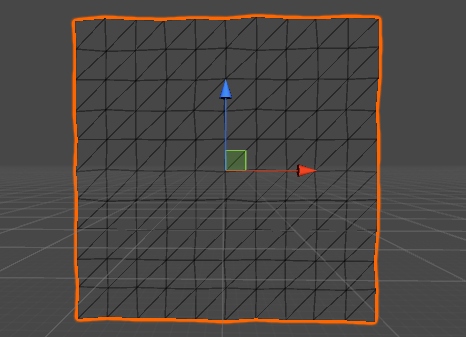### 1.渲染

• 如果你想要在Unity显示一些东西，你需要一个网格。他可以是一个3D模型从另一个程序倒入的（3dmax，maya）。它也可以是程序生成的网格。它可以是精灵、UI元素或者是粒子系统，它们一样都是使用unity网格，甚至是屏幕特效也是使用网格渲染的。
• 什么是网格？概念上来说网格由图形硬件（GPU Graphics Processing Unit图形处理单元）构成来绘制复杂的材料。它至少包含一组在3D空间中位置明确的点再加一组三角形构成。
• 最基础的2D图形，由点连接组成，面上的三角形就是此类网格的代表。
• 因为三角形是平坦的并且拥有直边，所以他们可以完美地被用来显示平坦的和连续的东西，像一个立方体的面孔。曲面的或者是圆的面只能被大量小的三角形来接近组成。如果三角面足够的小（不大于一个像素），那么你就不会感觉曲面和圆是由三角面组成的。从实时性能角度来讲通常这种情况是不可能的，所以我们总能够在面的某个程度上发现锯齿。
###### 仔细看下面的图你会发现，锯齿状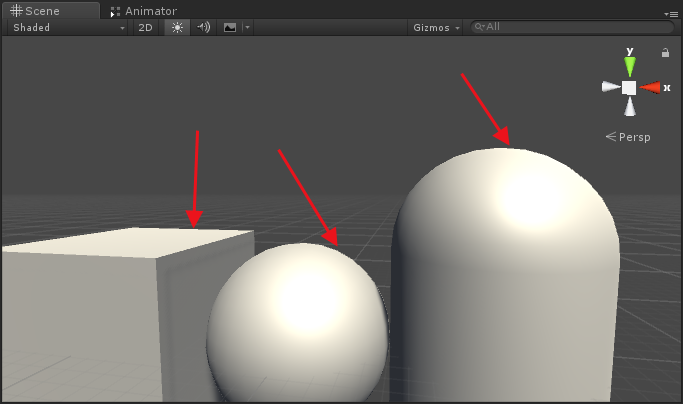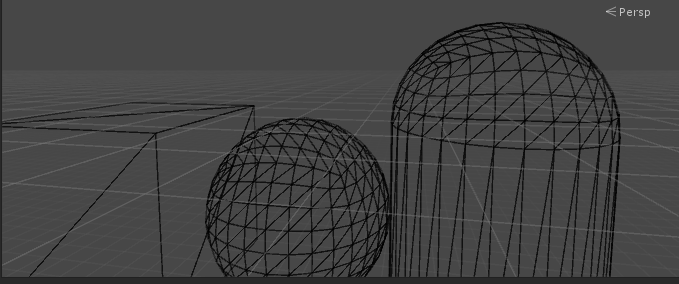Unity自带的胶囊体，立方体和球体 着色vs线框

Wireframe着色并带着线框

• 如果你想要展示一个3D游戏物体，它必须拥有两个组件。
• MeshFilter这个组件记录了你想要展示的网格数据
• MeshRenderer使用这个组件告诉网格如何渲染，比如使用哪个材质球，是否接受阴影和其他设置。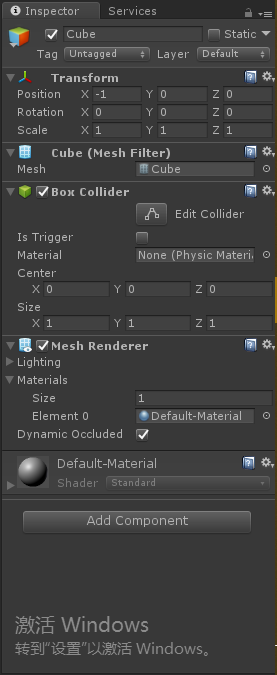• 一个快速添加细节的方法是给你的网格提供一个反射贴图，这个纹理描绘了材质球的基础颜色。当然我们需要知道如何投射纹理到网格的三角面上。这需要添加2D纹理坐标到定点上。这二维纹理空间被称为U和V，也是常说的UV坐标。UV坐标通常在（0,0）到（1,1）之间，它覆盖了整个纹理。超出范围的坐标将造成clamped或者Tiling平铺的效果，这取决于纹理设置。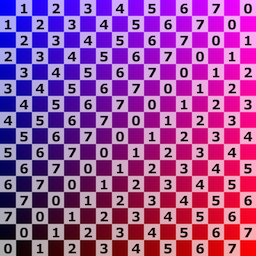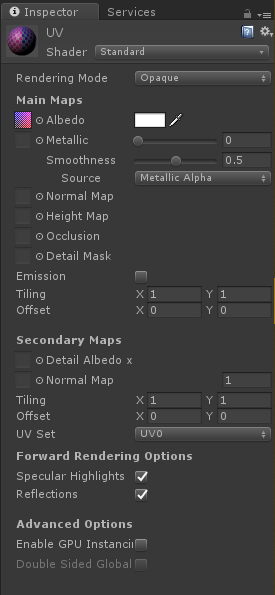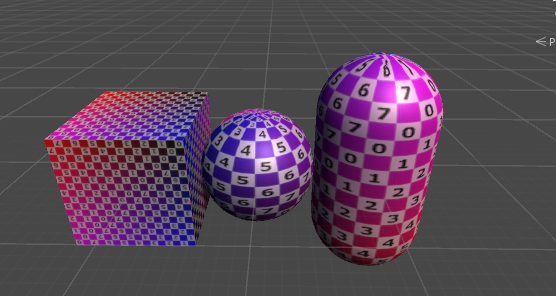### 2.创建网格顶点

``````using UnityEngine;
using System.Collections; //协程使用

//依赖组件（使用时当该脚本挂载到游戏对象身上会自动添加下面两个组件，作为该脚本的依赖项）
[RequireComponent(typeof(MeshFilter),typeof(MeshRenderer))]
public class Grid : MonoBehaviour  {

/// <summary>
/// 矩阵x，y位置
/// </summary>
public int xSize, ySize;
}
``````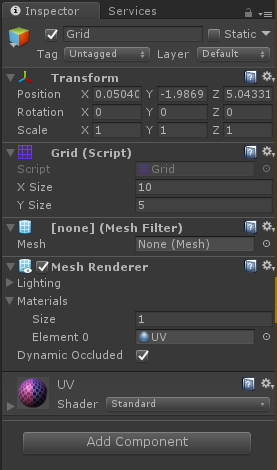• 现在我们先考虑定点位置稍后处理三角面序号。我们需要一个数组存储3D顶点位置。顶点的数量取决于网格的尺寸。我们需要获取每个四边形的顶点，但是相邻的四边形可以共享相同的顶点。所以每个维度上顶点数量比网格数多一个。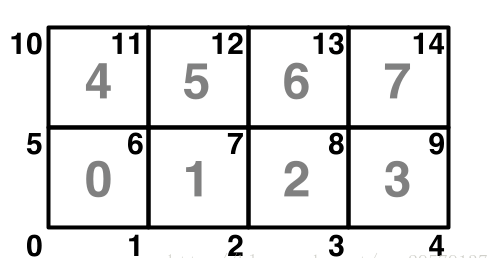###### 4 x 2网格
``````    /// <summary>
/// 网格顶点坐标
/// </summary>
private Vector3[] vertices;

private void Generate()
{
vertices = new Vector3[(xSize + 1) * (ySize + 1)];
}
``````
• 我们在场景中绘制这些顶点，这样我们就可以正确地核对他们的位置。利用OnDrawGizmos方法来挥之顶点的位置，利用OnDrawGizmos在每个顶点绘制一个小球。
``````    private void OnDrawGizmos()
{
Gizmos.color = Color.black;
if (vertices == null) return;
for (int i = 0; i < vertices.Length; i++)
{
Gizmos.DrawSphere(vertices[i],0.1f);
}
}
``````
• 什么是Gizmos？
• 在编辑器模式下Gizmos可以提供可视化的提示。他们只在Scene场景中显示不在PLay模式下显示，但是你可以通过工具栏调整它们。Gizmos公共类允许你绘制图表，线条，和其他的东西。
• Gizmos在OnDrawGizmos方法中执行绘制，它被Unity编辑器自动调用。另一个可选的方法是OnDrawGizmosSelected，他只能被可选的对象调用。
• 上面的没有画出来是因为Vector3数组为空，所以我们将其Generate方法修改一下
``````    private void Generate()
{
vertices = new Vector3[(xSize + 1) * (ySize + 1)];
for (int i = 0, y = 0; y <= ySize; y++)
{
for (int x = 0; x <= xSize; x++, i++)
{
vertices[i] = new Vector3(x, y);
}
}
}
``````
• 生成真实的网格在物理被唤醒的时候，Awake方法将在我们游戏运行模式时自动调用。
``````  private void Awake()
{
Generate();
}
``````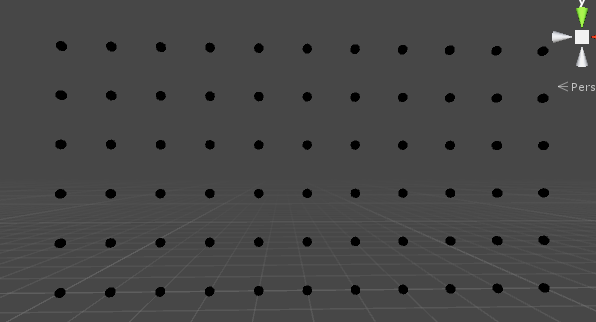##### 网格的顶点
• 为什么在Gizmos绘制不能被移动？
• Gizmos直接使用世界坐标绘制，不是使用对象的本地坐标系，如果你想遵循对象Transform组件，你必须声明使用transform.TransformPoint(vertices[i])代替vertices[i].
• 现在我们可以看见这些顶点，但是他们生成的顺序我们不能明显地看出来。我们可以使用颜色来标识，但是我们也可以使用协程减慢这个过程的速度。
观察顶点逐个生成

### 3.创建网格

• 现在我们知道顶点的位置是正确的，接下来我们就处理真正的网格。初次之外我们想要我们的组件持有这些顶点，我们必须要把顶点指定到MeshFilter.mesh中。我们处理过顶点之后，就可以网格存储在MeshFilter中。修改如下
``````
private Mesh mesh;

private IEnumerator Generate()
{
WaitForSeconds wait = new WaitForSeconds(0.05f);
GetComponent<MeshFilter>().mesh = mesh = new Mesh();
mesh.name = "Procedual Grid";
vertices = new Vector3[(xSize + 1) * (ySize + 1)];
for (int i = 0, y = 0; y <= ySize; y++)
{
for (int x = 0; x <= xSize; x++, i++)
{
vertices[i] = new Vector3(x, y);
yield return wait;
}
}

mesh.vertices = vertices;
}
``````
• 如何让组件持有网格？
• 大家可能发现MeshFilter组件的Mesh属性只有在Play模式下才能看得到，在Editor模式下是不存在的，这里我们需要如何才能将网格持久化保存呢。持久化
###### 网格只在Play模式下显示
• 到目前，在Play模式下我们可以持有一个网格，但是它依旧是不可见的，因为我们并没有给它任何三角面。三角面由定点数组索引决定。每一个三角面有三个顶点，三个连续的顶点绘制了一个三角形，让我们来先绘制第一个三角面。修改如下
``````    private IEnumerator Generate()
{
WaitForSeconds wait = new WaitForSeconds(0.05f);
GetComponent<MeshFilter>().mesh = mesh = new Mesh();
mesh.name = "Procedual Grid";
vertices = new Vector3[(xSize + 1) * (ySize + 1)];
for (int i = 0, y = 0; y <= ySize; y++)
{
for (int x = 0; x <= xSize; x++, i++)
{
vertices[i] = new Vector3(x, y);
yield return wait;
}
}

mesh.vertices = vertices;
DrawFaces(mesh);
}

/// <summary>
/// 绘制三角面
/// </summary>
private void DrawFaces(Mesh mh)
{
int[] triangles = new int;
triangles = 0;
triangles = 1;
triangles = 2;
mesh.triangles = triangles;
}
``````
• 这时候我们发现绘制了一个三角面，但是由于这三个顶点在一条直线上，所以生成了一个失败的三角形，它是不可见的一条线。如下图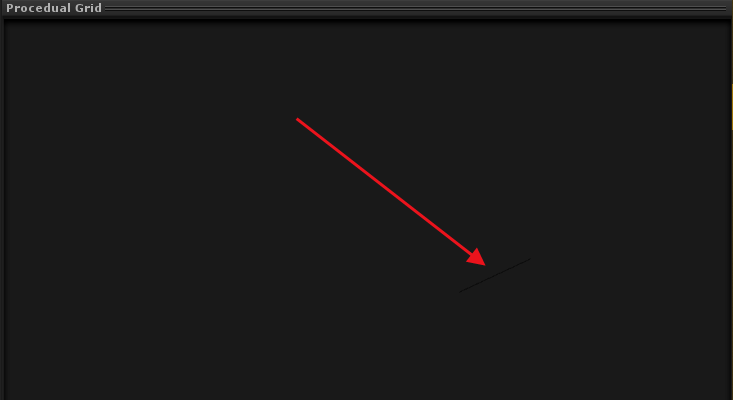• 第三个顶点我们将其转到下一行的第一个顶点。
``````        triangles = 0;
triangles = 1;
triangles = xSize + 1;
``````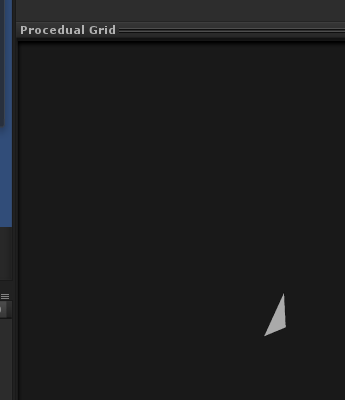• 通过以上操作，我们绘制了一个三角形，但是它只能在一个方向可见。这种情况下，只有Z轴的反方向可见，所以你可能需要旋转视角才能看得到。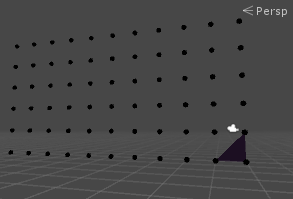• 三角形的哪一面可见是由定点序号的方向来确定的。默认情况下，如果定点顺序是顺时针方向的话那么三角形就是正面可见。逆时针（就是逆屏幕方向）的三角形是被抛弃的，所以我们不必花费时间去渲染这部分定点，以为他们通常都是不可见的。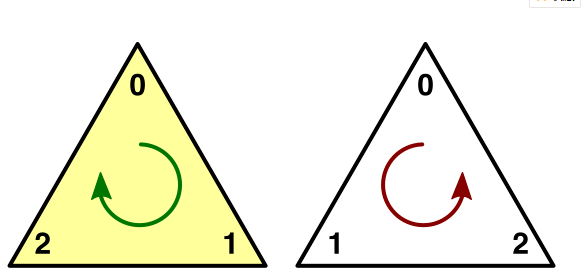###### 逆时针和顺时针三角形
• 所以为了实线从Z轴负方向到正方向可见，我们必须改变顺序相反的顶点的位置。我们交换后两个顶点的序号即可。
``````        triangles = 0;
triangles = xSize + 1;
triangles = 1;
``````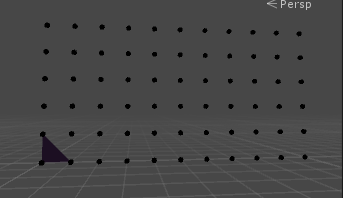###### 第一个三角面
• 现在我们绘制了一个三角面只覆盖了一个四方瓦片的一半，为了覆盖整个瓦片，我们需要第二个三角形面。
``````int[] triangles = new int;
triangles = 0;
triangles = xSize + 1;
triangles = 1;
triangles = 1;
triangles = xSize + 1;
triangles = xSize + 2;
``````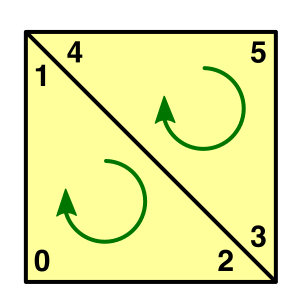###### 一个四边形由两个三角面组成
• 既然这些顶点共用两个顶点，我们就可以减少我们代码的行数，明确地提到每个顶点索引只有一次。
``````triangles = 0;
triangles = triangles = 1;
triangles = triangles = xSize + 1;
triangles = xSize + 2;
``````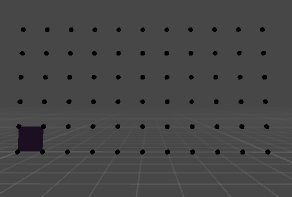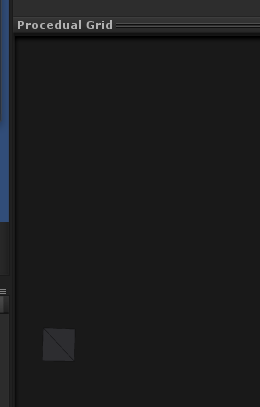###### 第一个四边形
• 我们可以通过循环来创建剩余第一行的瓦片。尽管我们遍历所有的顶点和三角面序号，但是我们必须要保证顶点和三角面序号是按照顺序的。我们把Yield代码的声明放到循环里，我们就不需要等待顶点的出现了。
``````
private IEnumerator Generate()
{
WaitForSeconds wait = new WaitForSeconds(0.05f);
GetComponent<MeshFilter>().mesh = mesh = new Mesh();
mesh.name = "Procedual Grid";
vertices = new Vector3[(xSize + 1) * (ySize + 1)];
for (int i = 0, y = 0; y <= ySize; y++)
{
for (int x = 0; x <= xSize; x++, i++)
{
vertices[i] = new Vector3(x, y);
yield return wait;
}
}

mesh.vertices = vertices;

int[] triangles = new int[6 * xSize];
for (int ti = 0, vi = 0, x = 0; x < xSize; x++, ti += 6, vi++)
{
triangles[ti] = vi;
triangles[ti + 3] = triangles[ti + 2] = vi + 1;
triangles[ti + 4] = triangles[ti + 1] = vi + xSize + 1;
triangles[ti + 5] = vi + xSize + 2;
yield return wait;
}
mesh.triangles = triangles;
yield return wait;
}
``````
• 现在，Gizmos可以立刻渲染出顶点，并且所有的三角面在一段时间后统一出现。要想看到瓦片一个接一个的出现，我们必须每次循环都刷新网格代替掉执行完所有循环刷新。
``````mesh.triangles = triangles;
yield return wait;
``````
###### 填充剩余网格
• 正如你所能看到的，所有的网格都被三角面填充，每一行都是同时填充，因为我们使用了协程。如果你对想过感到满意，你可以移除掉所有的协程代码，这样网格创建就没有任何的延迟了。
``````private void Awake ()
{
Generate();
}

private void Generate ()
{
GetComponent<MeshFilter>().mesh = mesh = new Mesh();
mesh.name = "Procedural Grid";

vertices = new Vector3[(xSize + 1) * (ySize + 1)];
for (int i = 0, y = 0; y <= ySize; y++) {
for (int x = 0; x <= xSize; x++, i++) {
vertices[i] = new Vector3(x, y);
}
}
mesh.vertices = vertices;

int[] triangles = new int[xSize * ySize * 6];
for (int ti = 0, vi = 0, y = 0; y < ySize; y++, vi++) {
for (int x = 0; x < xSize; x++, ti += 6, vi++) {
triangles[ti] = vi;
triangles[ti + 3] = triangles[ti + 2] = vi + 1;
triangles[ti + 4] = triangles[ti + 1] = vi + xSize + 1;
triangles[ti + 5] = vi + xSize + 2;
}
}
mesh.triangles = triangles;
}
``````
• 为什么不使用单个四边形?
• 当我们创建一个平面矩形，我们可以仅仅使用两个三角面。这没有问题。但是更多的顶点结构也可以提供更多的控制和表现。这里也只是一个实验!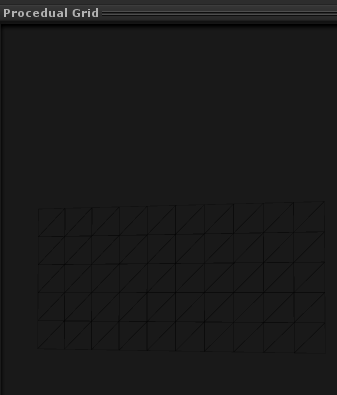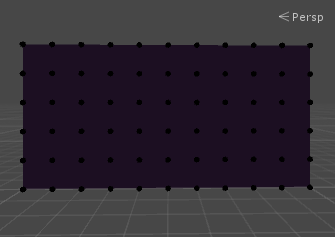### 4.生成额外的顶点数据：

unity3d 创建携程 相关内容